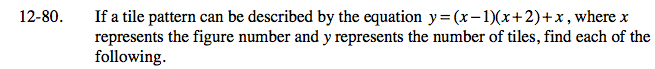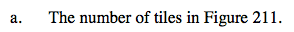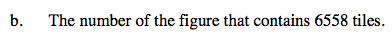### Home > AC > Chapter 12 > Lesson 12.4.2 > Problem12-80

12-80.Substitute 211 into the equation for x.Substitute 6558 into the equation for y.

6558 = (x − 1)(x + 2) + x

6558 = x² + x − 2 + x

6558 = x² + 2x + 2

Set the equation equal to zero.

0 = x² + 2x − 6556

Factor the expression.

0 = (x + 82)(x − 80)

x = −82 or 80

The figure number cannot be negative.

Figure 80